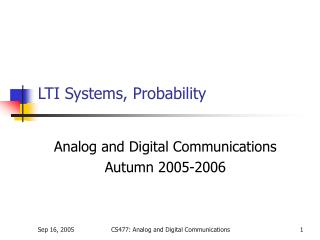# LTI Systems, Probability - PowerPoint PPT PresentationDownload PresentationLTI Systems, Probability

Download Presentation## LTI Systems, Probability

- - - - - - - - - - - - - - - - - - - - - - - - - - - E N D - - - - - - - - - - - - - - - - - - - - - - - - - - -
##### Presentation Transcript

1. LTI Systems, Probability Analog and Digital Communications Autumn 2005-2006 CS477: Analog and Digital Communications

2. Sampling • First consider modulation • Product with Cosine in time domain • Convolution with two impulses in frequency domain • Next consider sampling • Product with a train of impulses in time domain • Convolution with a train of impulses in the frequency domain • Nyquist sampling theorem • A bandlimited signal [-B, +B] can be characterized by its samples taken every 1/(2B) seconds. i.e., 2B samples per second • Undersampling leads to aliasing CS477: Analog and Digital Communications

3. LTI Systems • Linearity If then • Time invariance • Linearity and Time invariance CS477: Analog and Digital Communications

4. Response of LTI Systems Impulse response: CS477: Analog and Digital Communications

5. Exponentials and LTI Systems Exponentials are eigenfunctions of LTI systems! LTI Systems can not generate new frequencies! CS477: Analog and Digital Communications

6. Hilbert Transformer • A filter introducing a constant delay of 90 degrees to the input signal • Hilbert transform does not change the domain; It’s merely a convolution CS477: Analog and Digital Communications

7. Random Variables • Outcomes and sample space • Random Variables • Mapping outcomes to: • Discrete numbers  Discrete RVs • Real line  Continuous RVs • Cumulative distribution function • One variable • Joint cdf CS477: Analog and Digital Communications

8. Random Variables • Probability mass function (discrete RV) • Probability density function (cont. RV) • Joint pdf of independent RVs • Mean • Variance • Characteristic function • (IFT of pdf) CS477: Analog and Digital Communications